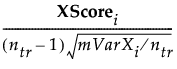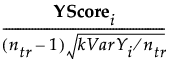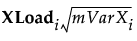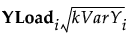Publication date: 07/30/2020

Consider the following notation:

ntr is the number of observations in the training set

m is the number of effects in X

k is the number of responses in Y

VarXi is the percent variation in X explained by the ith factor

VarYi is the percent variation in Y explained by the ith factor

XScorei is the vector of X scores for the ith factor

YScorei is the vector of Y scores for the ith factor

### Standardized Scores

The vector of ith Standardized X Scores is defined as follows:The vector of ith Standardized Y Scores is defined as follows: